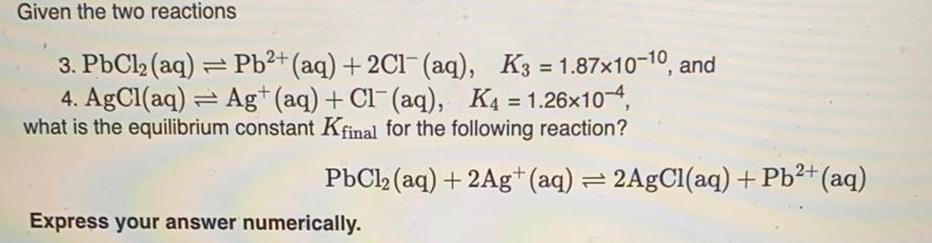Question:

# Given the two reactions 3. PbCl₂ (aq) = Pb²+ (aq) + 2Cl¯

Last updated: 8/5/2022Given the two reactions 3. PbCl₂ (aq) = Pb²+ (aq) + 2Cl¯ (aq), K3=1.87x10-10, and 4. AgCl(aq) Ag+ (aq) + Cl(aq), K4 = 1.26x10-4, what is the equilibrium constant Kfinal for the following reaction? PbCl₂ (aq) + 2Ag+ (aq) = 2AgCl(aq) + Pb²+ (aq) Express your answer numerically.